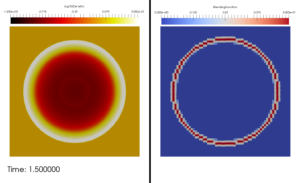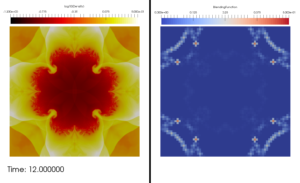# Snapshot: Blast wave simulation computed in FLUXO with an entropy-stable high-order Discontinuous Galerkin/Finite Volume hybrid scheme

Blast wave simulation with periodic boundaries computed in FLUXO with an
entropy-stable high-order Discontinuous Galerkin/Finite Volume scheme.The scheme is formulated in Hennemann and Gassner. “A provably entropy stable subcell shock capturing approach for high order split form DG.” Journal of Computational Physics (submitted). The scheme computes the spatial operator as F = (1-a) F_{DG} + a F_{FV}, where a is the blending function